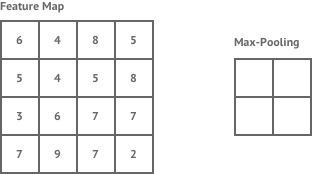# A Convolutional Net in 30 Lines of Code

Just give me the code:

``````from keras.preprocessing.image import ImageDataGenerator
from keras.models import Sequential
from keras.layers import Conv2D, MaxPool2D, Dense, Flatten

# create model
model = Sequential()
model.add(Conv2D(32, (5, 5), activation="relu", input_shape=(256, 256, 3)))

# get data
train_gen = ImageDataGenerator(rotation_range=0.3, rescale=1/255, shear_range=0.2, zoom_range=0.2, horizontal_flip=True)
test_gen = ImageDataGenerator(rescale=1/255)

train_set = train_gen.flow_from_directory("Dataset Fussbaler/Training Set", batch_size=32)
test_set = test_gen.flow_from_directory("Dataset Fussbaler/Test Set", batch_size=32)

# train model
model.fit_generator(train_set, steps_per_epoch=1000, epochs=1, validation_data=test_set)

model.save("Keras_models/class_conv_v1")``````

Hello World! I’m Peer.

This is my first post and it will be about a Convolutional Neural Network. In this article, I will call this neural network CNN. I will start by talking about the rough features of a CNN . Then I will talk about the implementation. We will be using Keras for the implementation and we’ll be classifying soccer players.https://leonardoaraujosantos.gitbooks.io/artificial-inteligence/content/moreimages/Convolutionschematic.gif

The convolutional operation “moves” through the image and multiplies the filter, with the part of the image, it is applied to, and then sums it up. Through that, the image size is reduced. This is helpful, as one convolution filter could look for the eyes of a person and another one could look for the nose if you would like to classify a person.https://leonardoaraujosantos.gitbooks.io/artificial-inteligence/content/imagefolder4/Relu.jpeg

The relu function is really simple. It is an activation function, just like sigmoid and tanh. It takes the maximum of its input and 0 and then returns it.https://shafeentejani.github.io/assets/images/pooling.gif

Max polling reduces the size of an image and reduces the amount of computation needed. It takes out of an area the maximum value and then puts it in a new image. This is good because most features are still in the image, however, it is much smaller.

The classification part is just a standard feed-forward neural network. At the beginning of the classification part is a layer which flattens the input of the feature learning part. In other words, it converts the input to a 1D array.

# The Implementation

At first, you need to install Keras and Tensorflow. Just visit their homepages and install them.

In the first 3 lines of code, we import Keras. We import an ImageDataGenerator, to easily import data out of our Dataset. Then we import a Sequential model, which is our network and provides an easy way to add layers and train. Then we import the layers we need.

In lines 5 - 16 we build our model. We start out with a convolutional layer with 32 5 by 5 filters. and follow it with a MaxPool layer with a filter size of 4 by 4. Then we add this pair one more time and add another combo of convolution and max-pooling, just this time with different parameters. All of those parameters where just took some what random and you could probably get better results than me, by just playing with those. Then we add the classification section, with a softmax activation function at the out layer.

We use the Adam optimizer and the categorical cross-entropy cost function.

In lines 21 - 25 we get our data. We use an ImageDataGenerator. Our Images are RGB and are 256 by 256 pixels big. To know more about ImageDataGenerators and generally about the functions used in this tutorial go to keras.io.

Then we train our network based on our dataset. We train it for 1000 steps, where it is trained on a mini batch, with the size of 32. Then we just need to save it. I used around 80 Images per person to train the CNN. The soccer players I used were Cristiano Ronaldo, Toni Kroos, Lionel Messi, Thomas Müller. After 1000 epochs I got about 85 % accuracy on the training data and about 90 % on the validation data.

# Conclusion

To improve this model I would suggest, to get more data and play around with the hyper parameters. However, I think the model worked out quite good, despite having only few training data and only training for 1000 steps. I encourage you to play around with this code and test it on your own data.